### 二、一阶线性方程

1    一阶齐次线性方程

[特征方程 特征曲线 初积分(首次积分)]  给定一阶齐次线性方程

`(1)`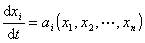( i = 1,2n )

`(2)`

如果函数( x1 , x2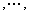xn )在特征曲线上等于常数，即( x1(t) , x2(t)xn(t) ) = c

[齐次方程的通解]

1o  连续可微函数u =( x1, x2xn ) 是齐次线性方程(1)的解的充分必要条件是：( x1, x2xn )是这个方程的特征方程的初积分.

2oi ( x1 , x2xn )  ( i = 1,2n) 是特征方程(2)在区域D上连续可微而且相互独立的初积分(因此在D内的每一点，矩阵u =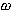(1 ( x1 , x2xn )n-1 ( x1 , x2xn ) )

[柯西问题考虑方程的柯西问题i ( x1 , x2xn )  ( i = 1,2n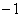) 为特征方程的任意n个相互独立的初积分，引入参变量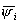(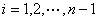)，从方程组u =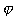(2 (1 ,2n-1 )n (1 ,2n-1 ) )